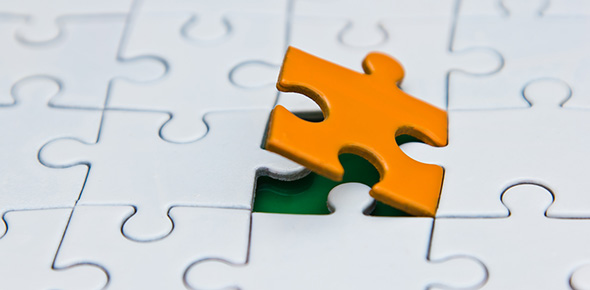Ll CSET 110 - Number Theory Mc

15 Questions | Total Attempts: 61Settings• 1.
5,078 = 150(33)+128 is the first step in finding the greatest common divisor of two numbers. Which of the following is the next step in the Euclidian Algorithm?
• A.

150=33(4)+18

• B.

128=33(3)+29

• C.

150=128(1)+22

• D.

5,078=128(39)+86

• 2.
The Euclidian Algorithm is performed to find the greatest common divisor of 1,622 and 136. Which of the following is a step in this Euclidian Algorithm?
• A.

136 = 11(12)+4

• B.

126=15(8)+6

• C.

126=12(10)+6

• D.

64=8(8)+0

• 3.
If  can be simplified to a fraction form , where a and b are mutually prime integers, what is a+b?
• A.

76

• B.

81

• C.

100

• D.

300

• 4.
If a and b share a greatest common divisor of c, what is the least common multiple of a and b?
• A.

Abc

• B.

Ab

• C.
• D.
• 5.
If  can be simplified to a fraction form , where a and b are mutually prime integers, what is a+b?
• A.

98

• B.

109

• C.

126

• D.

327

• 6.
Let P represent the set of all numbers of the form  where x, y, and z are prime numbers less than 20. How many elements are contained in set P ?
• A.

56

• B.

512

• C.

729

• D.

6561

• 7.
How many factors does  have if x, y, and z are integers?
• A.

Xyz

• B.

X+y+z

• C.

(x+1)(y+1)(z+1)

• D.

105

• 8.
The greatest common divisor of . How many factors of X are there?
• A.

12

• B.

16

• C.

24

• D.

36

• 9.
What is the greatest common divisor of 4986, 5022, 5058, and 5490?
• A.

6

• B.

9

• C.

12

• D.

18

• 10.
Find the least common multiple of 12, 18, 24, and 30.
• A.

240

• B.

360

• C.

720

• D.

2160

• 11.
Find the least common multiple of 18, 27, 36, 48, 81.
• A.

1260

• B.

1440

• C.

1620

• D.

4860

• 12.
How many integers between 1 and 1000, inclusive, are a multiple of either 3 or 7?
• A.

381

• B.

428

• C.

430

• D.

475

• 13.
How many integers between 1 and 1000, inclusive, are a multiple of either 5 or 9?
• A.

288

• B.

289

• C.

311

• D.

312

• 14.
To prove that  , the first step in a proof by mathematical induction is which of the following?
• A.

is also true.

• B.
• C.
• D.
• 15.
has a positive integer exponents and  is between 4000 and 5000, which of the following could be x+y+z?
• A.

6

• B.

9

• C.

11

• D.

15Back to top